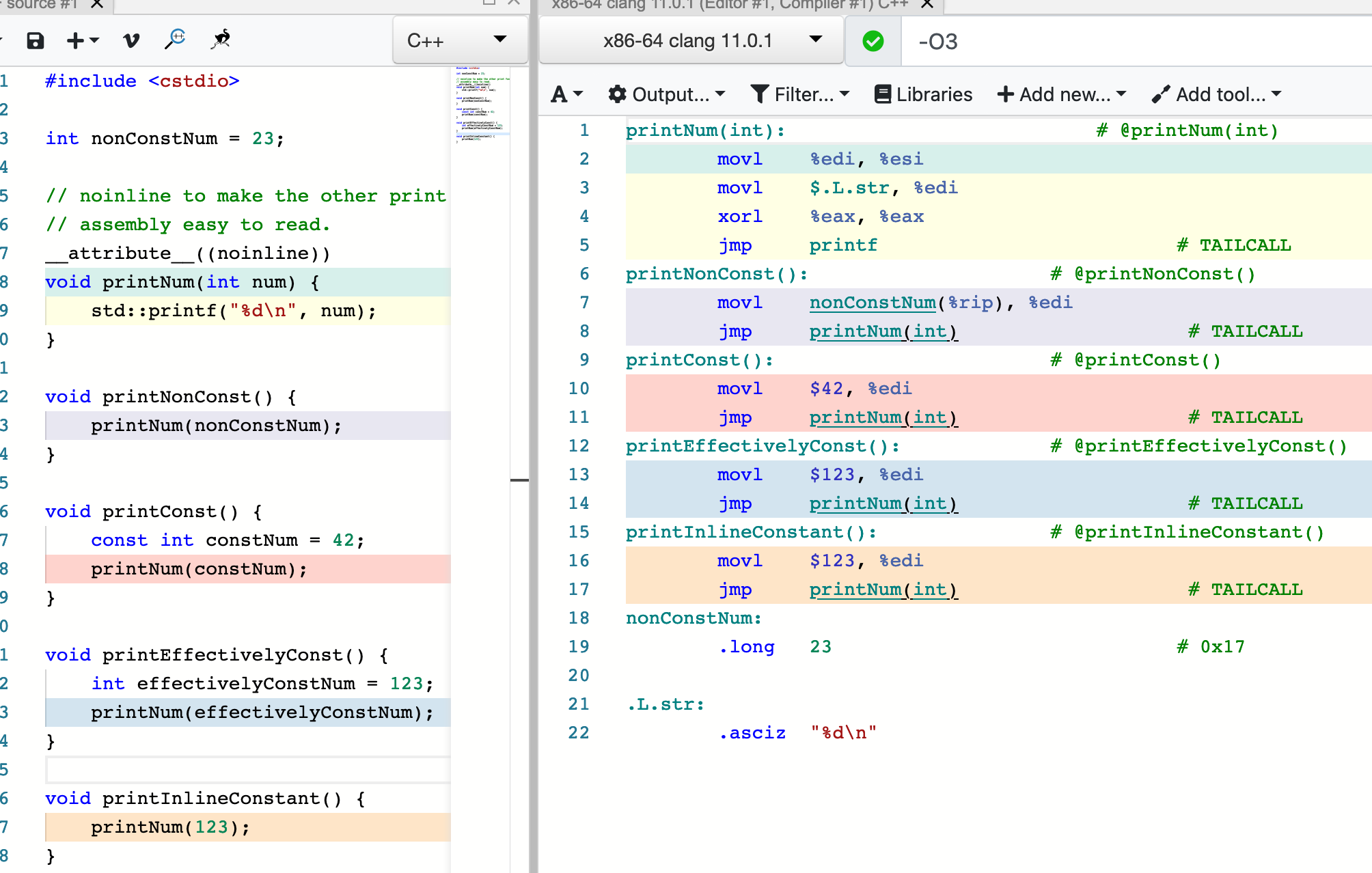void multiplyArrayByTwoConstants(std::span<int> arr, int x, int y) {
for (int& element: arr) {
element *= x * y;
}
}


x*y和循环没关系，会被外提到for之前算好

### inline + constant propagation

inline + const出现的路径都会优化成立即数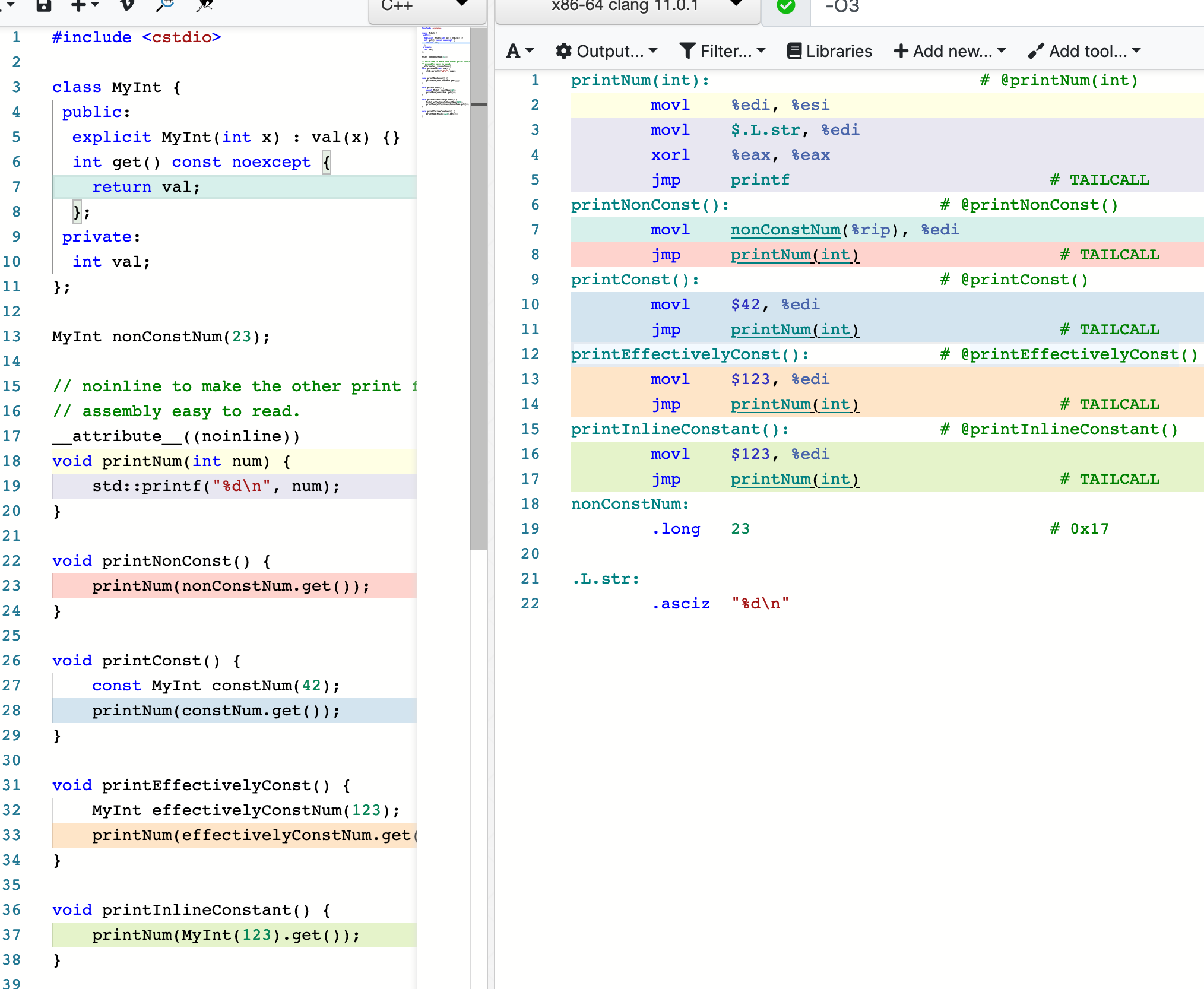### inline + virtual + constant propagation

class MyInt {
public:
explicit MyInt(int x) : val(x) {}
virtual int get() const noexcept {
return val;
};
private:
int val;
};

MyInt nonConstNum(23);

// noinline to make the other print functions'
__attribute__((noinline))
void printNum(int num) {
std::printf("%d\n", num);
}

void printArgByValue(MyInt num) {
printNum(num.get());
}

void printArgByConstReference(const MyInt& num) {
printNum(num.get());
}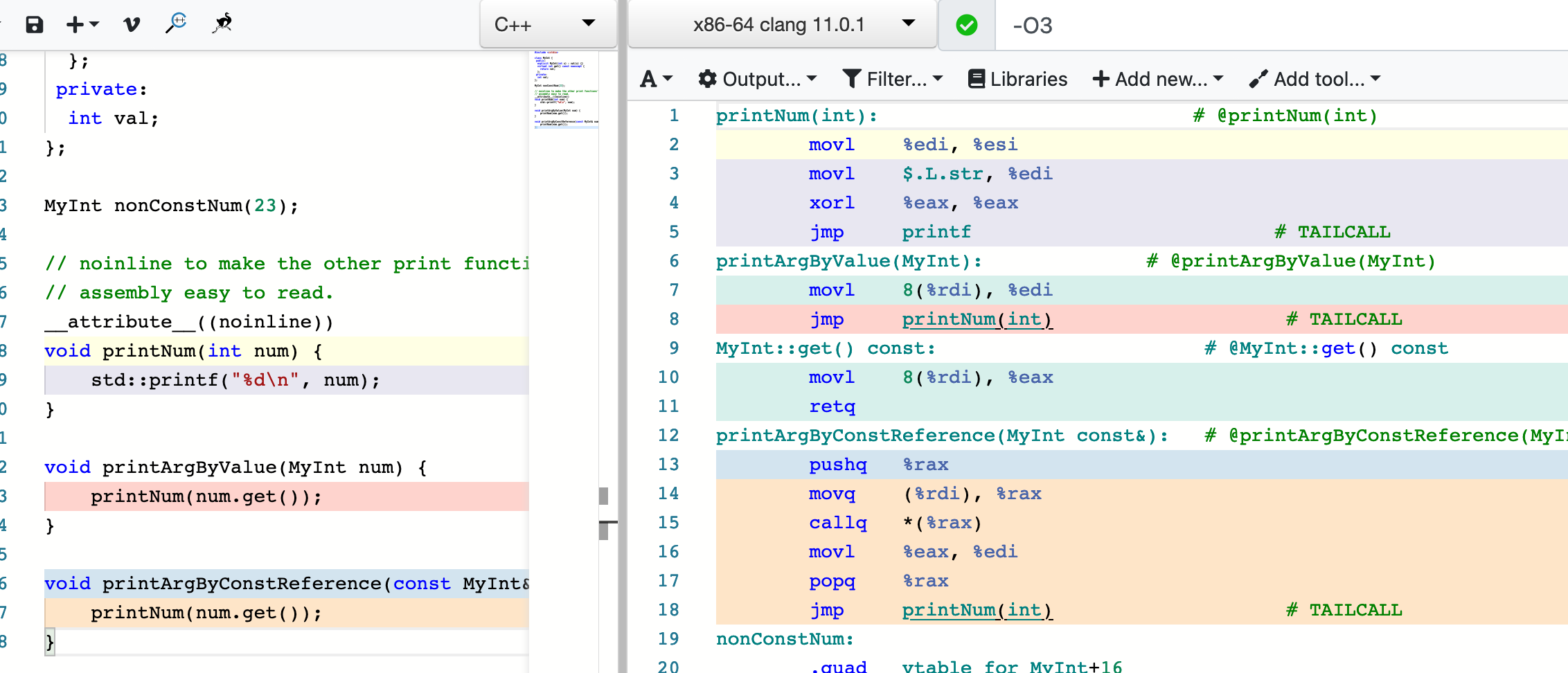### inline + LICM

class MyInt {
public:
explicit MyInt(int x) : val(x) {}
int get() const noexcept {
return val;
};
private:
int val;
};

void multiplyArrayByTwoConstants(std::span<int> arr, MyInt x, MyInt y) {
for (int& element: arr) {
element *= x.get() * y.get();
}
}


### 一个经典LICM例子 strlen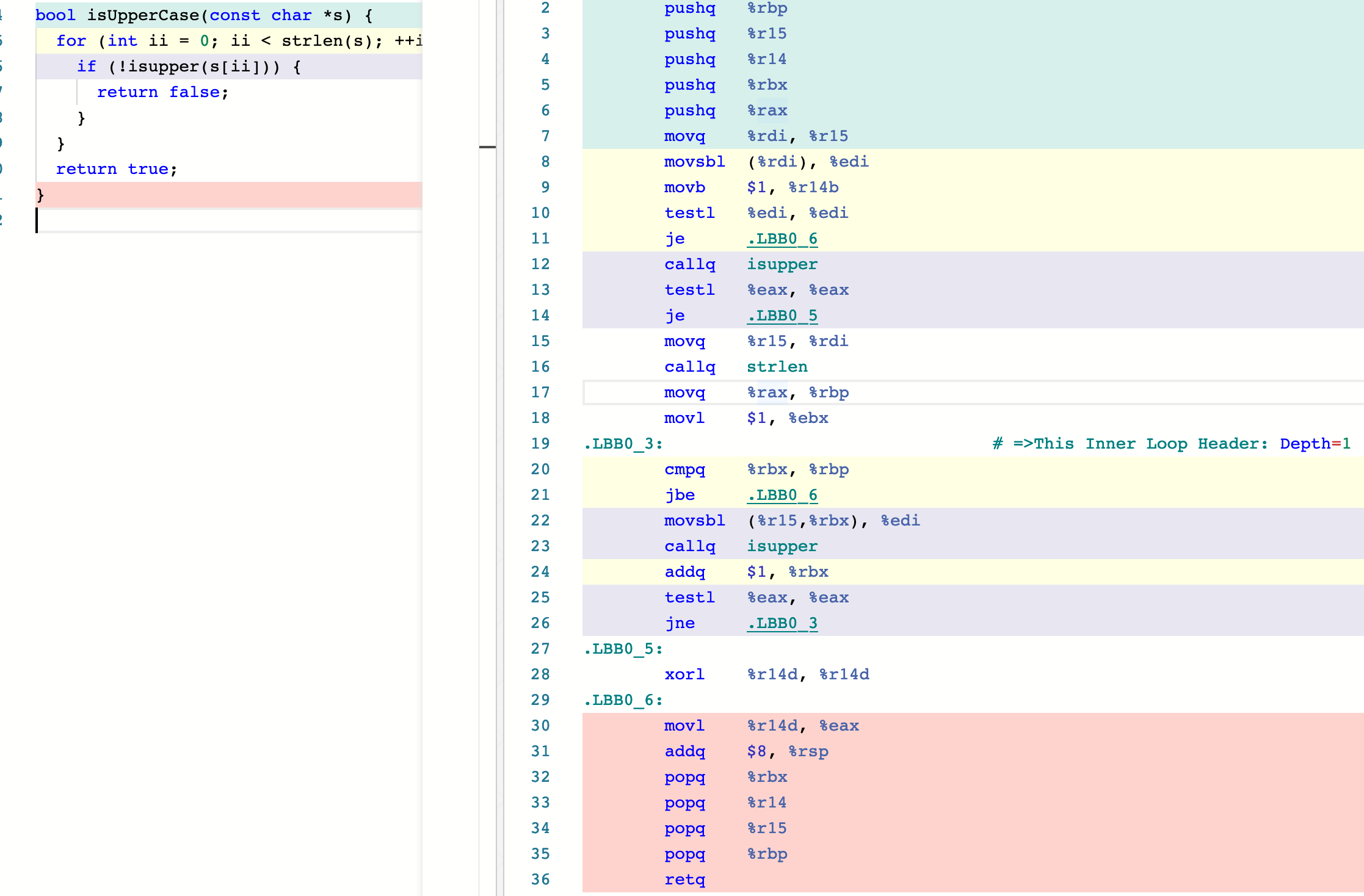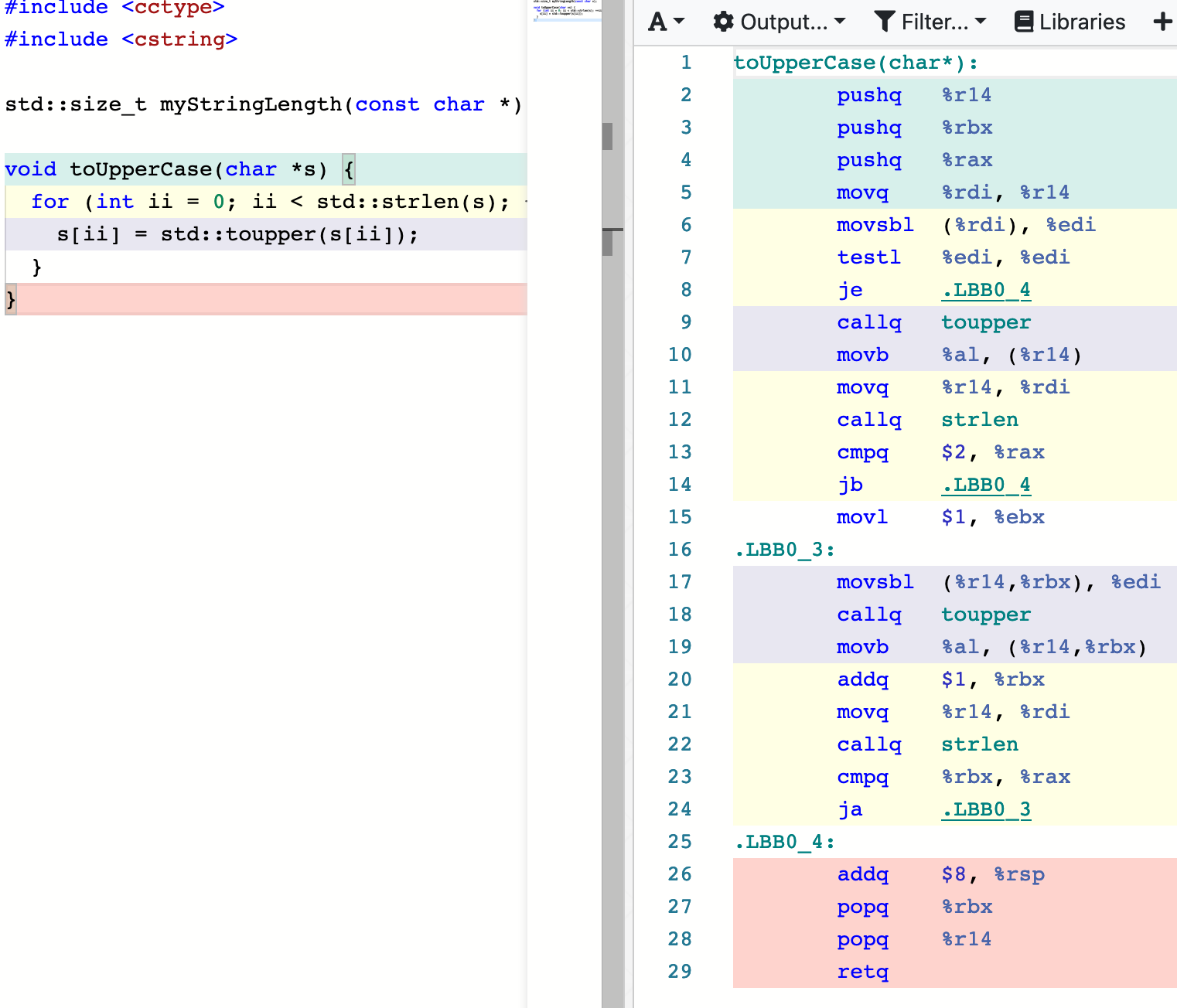#include <cctype>
#include <cstring>

void toUpperCase(char *s) {
while (*s) {
*s = std::toupper(*s);
s++;
}
}